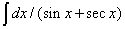# please give me the solution for this question as soon as possible8 years ago

Dear student,

v3 cosec20 – sec 20

v3/sin20-1/cos20

=[v3cos20-sin20]/[sin20cos20]

=2[v3/2.cos20 -1/2 sin20]/sin 20cos20

=2[cos30.cos20-sin30.sin20]/sin20cos20

=2[cos(20+30)]/[sin20cos20]

=2os50/sin20cos20

=4cos50/2sin20cos20

=4cos50/sin40

=4cos(90-40)/sin40

=4sin40/sin40

=4

Thanks & Regards

Rinkoo Gupta